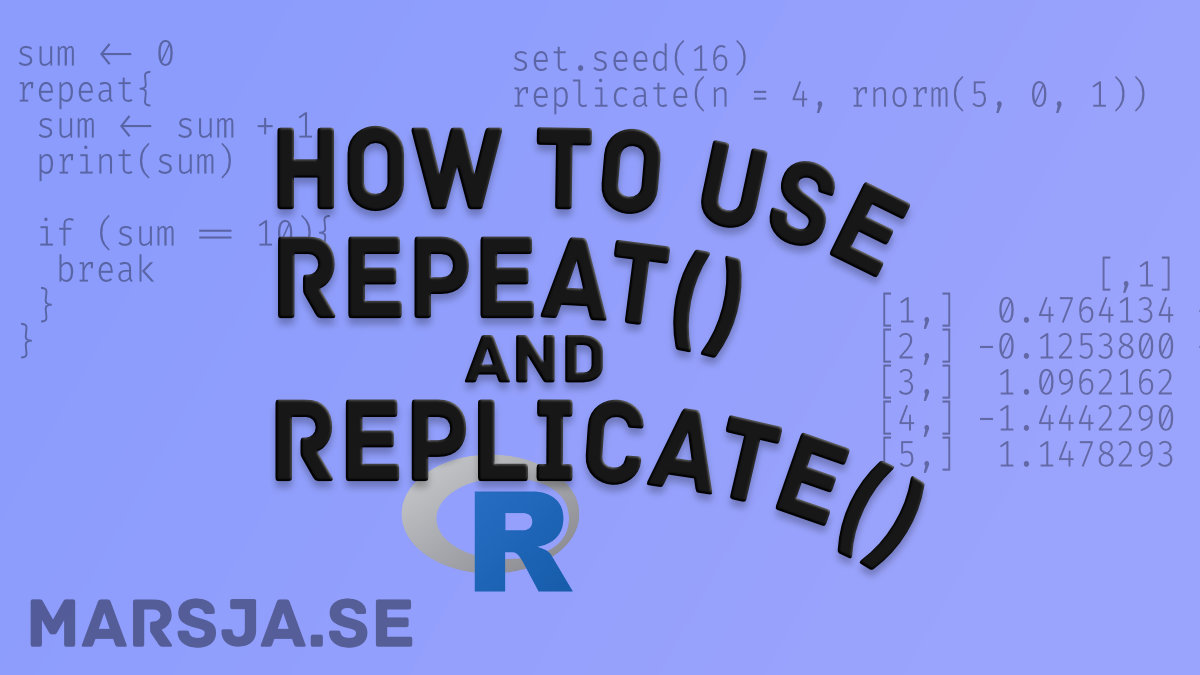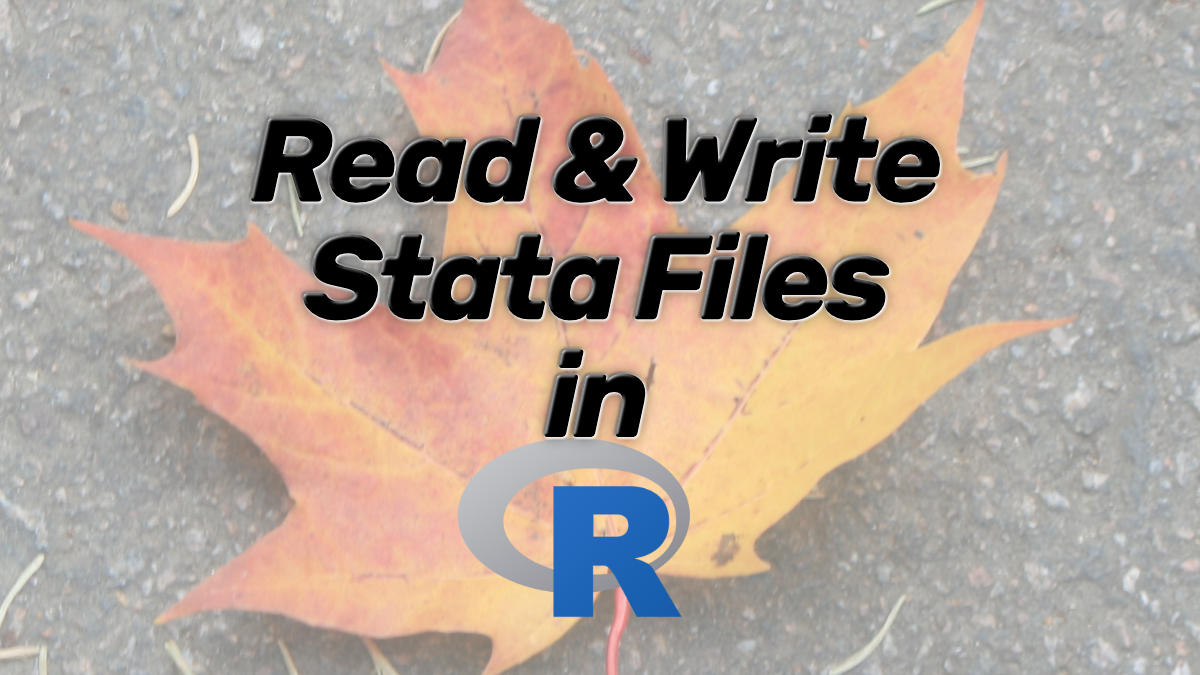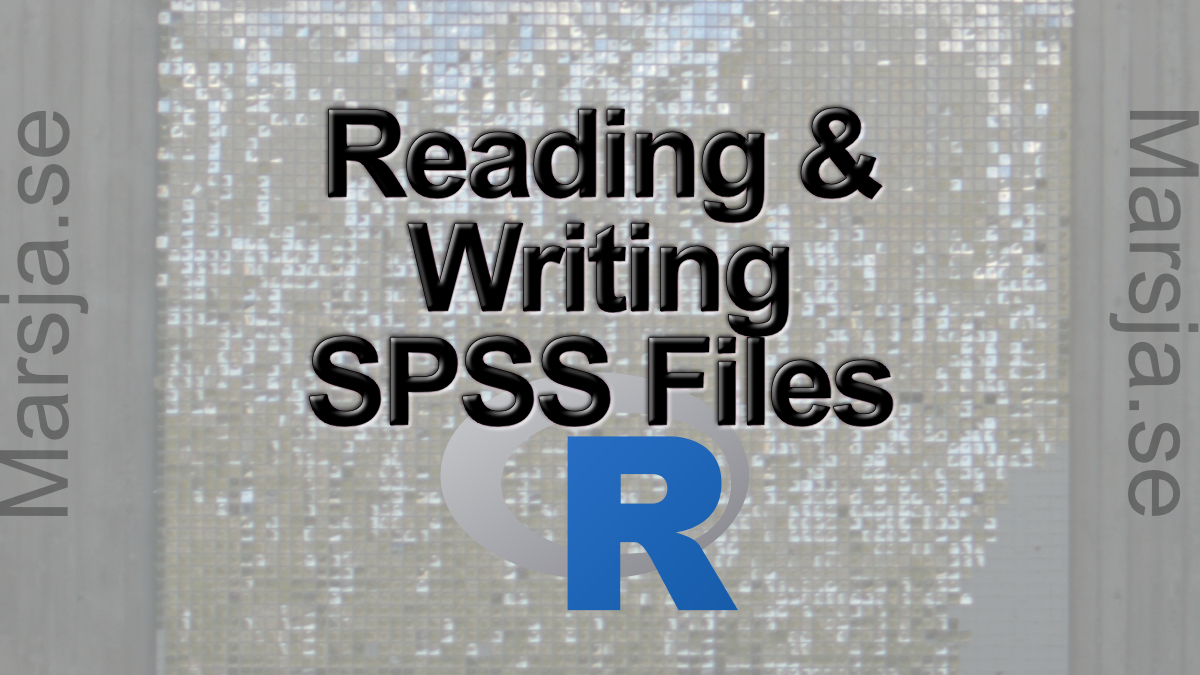## How to use the Repeat and Replicate functions in R

In this short tutorial, we will learn how to use the repeat and replicate functions in R. These two functions, repeat and replicate, are two very useful functions. Repeat and Replicate Now, before we learn how to use both repeat and replicate, we will answer two...## How to Read and Write Stata (.dta) Files in R with Haven

In this post, we are going to learn how to read Stata (.dta) files in R statistical environment. Specifically, we will learn 1) who to import .dta files in R using Haven, and 2) how to write dataframes to .dta file. Data Import in R: Reading Stata Files Now, R is, as...## How to Remove a Column in R using dplyr (by name and index)

In this remove a column in R tutorial, we are going to work with dplyr to delete a column. Here, we are going to learn how to remove columns in R using the select() function. Specifically, we are going to remove columns by name and by index. Finally, we will also...## Learn How to Calculate Descriptive Statistics in R the Easy Way

In this post, we will learn how to carry out descriptive statistics in R. After we have learned how to do this, we will learn how to create a nice latex table and how to save the summary statistics to a .csv file. Why Descriptive Statistics? Carrying out descriptive...## How to Read & Write SPSS Files in R Statistical Environment

In this post we are going to learn 1) how to read SPSS (.sav) files in R, and 2) how to write to SPSS (.sav) files using R.  More specifically, here we are going to work with the following two R packages haven (from the Tidyverse) and foreign to: Read a .sav file...